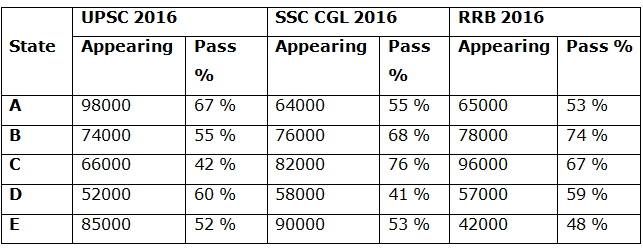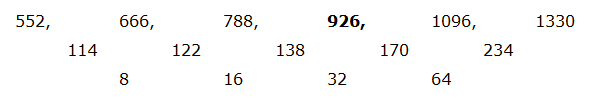# CWC/FCI Prelims 2019 – Quantitative Aptitude Questions (Day-01)

Dear Readers, Exam Race for the Year 2019 has already started, To enrich your preparation here we are providing new series of Practice Questions on Quantitative Aptitude – Section for CWC/FCI Exam. Aspirants, practice these questions on a regular basis to improve your score in aptitude section. Start your effective preparation from the right beginning to get success in upcoming CWC/FCI Exam.

[WpProQuiz 5384]

Directions (Q. 1 – 5): Find the wrong term in the following number series?

1) 772, 1397, 495, 1722, 122, 2147

a) 1397

b) 495

c) 1722

d) 2147

e) 772

2) 9, 13, 35, 122, 509, 2581

a) 13

b) 2581

c) 35

d) 122

e) 509

3) 657, 656, 327, 108, 25

a) 25

b) 327

c) 657

d) 108

e) 656

4) 53, 26, 27, 39, 80, 197, 595.5

a) 26

b) 53

c) 197

d) 595.5

e) 39

5) 552, 666, 788, 924, 1096, 1330

a) 924

b) 666

c) 1096

d) 1330

e) 788

Directions (Q. 6 – 10): Study the following information carefully and answer the given questions

The following table shows the total number of students appearing for different examination and the percentage of students passed in the tier – 1 examination from different states.6) Total number of students appearing from A and B together is approximately what percentage of total number of students appearing from D and E together for all the given examination?

a) 96 %

b) 84 %

c) 118 %

d) 102 %

e) 135 %

7) Find the total number of students passed in the UPSC 2016 tier – 1 for all the given states together?

a) 178560

b) 209480

c) 165270

d) 214530

e) None of these

8) Total number of passed students of UPSC and RRB 2016 tier – 1 in State A, C and E together is approximately what percentage more/less than the total number of passed students of SSC CGL and RRB 2016 tier – 1  in State B, D and E together?

a) 9 % more

b) 14 % less

c) 27 % more

d) 14 % more

e) 9 % less

9) Find the ratio between the total number of appearing students of SSC CGL 2016 to that of UPSC 2016 tier – 1?

a) 32: 35

b) 47: 49

c) 51: 53

d) 74: 75

e) None of these

10) Find the sum of the total number of students passed the tier – 1 examination from state C of all the given examination?

a) 172430

b) 154360

c) 218950

d) 245270

e) None of these

Direction (1-5) :

The correct series is,

772, 1397, 497, 1722, 122, 2147

The pattern is, +252, -302, +352, -402, +452,…..

The wrong term is, 495

The correct series is,

9, 13, 35, 121, 509, 2581

The pattern is, *1+22, *2+32, *3+ 42, *4+ 52, *5+ 62,….

The wrong term is, 122

The correct series is,

657, 656, 327, 108, 26

The pattern is,

(657-1)/1 = 656, (656 – 2)/2 = 327, (327-3)/3 = 108, (108-4)/4 = 26

The wrong term is, 25

The correct series is,

53, 26, 27, 39, 80, 197.5, 595.5

The pattern is, *0.5 – 0.5, *1 + 1, *1.5 -1.5, *2 + 2, *2.5 – 2.5, *3 + 3,…

The wrong term is, 197

The correct series is,The difference of difference is, ×2

The wrong term is, 924

Direction (6-10) :

Total number of students appearing from A and B together for all the given examination

= > 98000 + 64000 + 65000 + 74000 + 76000 + 78000

= > 455000

Total number of students appearing from D and E together for all the given examination

= > 52000 + 58000 + 57000 + 85000 + 90000 + 42000

= > 384000

Required % = (455000/384000)*100 = 118.48 % = 118 %

Total number of students passed in the UPSC 2016 tier – 1 for all the given states together

= > 98000(67/100) + 74000*(55/100) + 66000*(42/100) + 52000*(60/100) + 85000*(52/100)

= > 65660 + 40700 + 27720 + 31200 + 44200

= > 209480

Total number of passed students of UPSC and RRB 2016 tier – 1 in State A, C and E together

= > 98000*(67/100) + 66000*(42/100) + 85000*(52/100) + 65000*(53/100) + 96000*(67/100) + 42000*(48/100)

= > 65660 + 27720 + 44200 + 34450 + 64320 + 20160

= > 256510

Total number of passed students of SSC CGL and RRB 2016 tier – 1 in State B, D and E together

= > 76000*(68/100) + 58000*(41/100) + 90000*(53/100) + 78000*(74/100) + 57000*(59/100) + 42000*(48/100)

= > 51680 + 23780 + 47700 + 57720 + 33630 + 20160

= > 234670

Required % = [(256510 – 234670)/234670]*100 = 9.306 % = 9 % more

The total number of appearing students of SSC CGL 2016 tier – 1

= > 64000 + 76000 + 82000 + 58000 + 90000

= > 370000

The total number of appearing students of UPSC 2016 tier – 1

= > 98000 + 74000 + 66000 + 52000 + 85000

= > 375000

Required ratio = 370000: 375000 = 74: 75